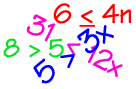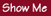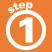Home    |    Teacher    |    Parents    |    Glossary    |    About UsJust like with equations, the solution to an inequality is a value that makes the inequality true. You can solve inequalities in the same way you can solve equations, by following these rules.Watchout! If you multiply or divide both sides of an inequality by a negative number, reverse the direction of the inequality sign!Homework Help | Algebra | Equations and InequalitiesEmail this page to a friendSearch·  Solving addition and     subtraction equations·  Multiplication equations·  Division equations·  Inequalities·  Formulas·  Two-step equations     and inequalitiesFirst Glance In Depth Examples WorkoutInequalities